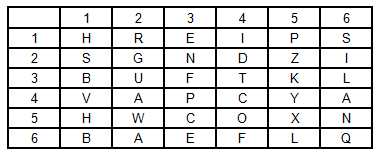Question 200

# In the following question, a word is represented by a set of numbers as given in any one of the alternatives. The sets of numbers given in the alternatives are represented by alphabets as in the matrices given below. The columns and rows of matrix are numbered from 1 to 6. A letter from these matrices can be represented first by its row and next by its column number, e.g., ‘A’ can be represented by 42, ‘S’ can be represented by 21, etc. Similarly, you have to identify the set for the word ‘PLAY’.Solution

as can be seen from the table, the alphabets and the numbers corresponding to them are:
P:43
L:65
A:62
Y:45
hence the correct answer is option D

• Free SSC Study Material - 18000 Questions
• 230+ SSC previous papers with solutions PDF